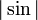# Absolute value of sine function

This article is about a particular function from a subset of the real numbers to the real numbers. Information about the function, including its domain, range, and key data relating to graphing, differentiation, and integration, is presented in the article.
View a complete list of particular functions on this wiki

## Definition

This function of one variable, denoted$|\sin|$, is defined as the composite of the absolute value function and the sine function, i.e., it is defined as:$x \mapsto |\sin x|$

The function can also be defined using a piecewise definition as follows:$|\sin x| = \left\lbrace \begin{array}{rl}\sin x, & 2n\pi \le x \le (2n + 1)\pi, n \in \mathbb{Z}\\ -\sin x, & (2n - 1)\pi < x < 2n\pi, n \in \mathbb{Z}\\\end{array}\right.$

## Key data

Item Value
default domain all real numbers, i.e., all of$\R$
range the closed interval$[0,1]$, i.e.,$\{ y : 0 \le y \le 1 \}$
absolute maximum value: 1, absolute minimum value: 0
period$\pi$
important symmetries even function (see composite of even function and odd function is even)
The function has mirror symmetry with respect to vertical lines for$x$-values at all multiples of$\pi/2$, including the even and odd multiples.
local maximum values and points of attainment local maximum value of 1, attained at all odd integer multiples of$\pi/2$
local minimum values and points of attainment local minimum value of 0, attained at all integer multiples of$\pi$ (or equivalently, all even integer multiples of$\pi/2$)
first derivative$x \mapsto \left\lbrace \begin{array}{rl}\cos x, & 2n\pi < x < (2n + 1)\pi, n \in \mathbb{Z}\\ -\cos x, & (2n - 1)\pi < x < 2n\pi, n \in \mathbb{Z}\\\end{array}\right.$ The function is not differentiable at multiples of$\pi$. At any multiple of$\pi$, the left hand derivative is -1 and the right hand derivative is +1.
second derivative$x \mapsto -|\sin x|$ except at integer multiples of$\pi$, where the second derivative is undefined.